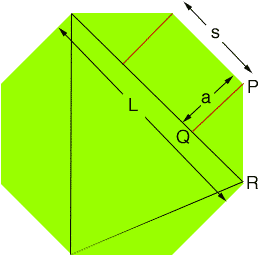Quandaries and Queries Name: Billy Question: How can you find the length of the side of an octagon when all that you know are the long sides of one of the eight perfect isc. triangles inside the octagon that share the same center point? Hi Billy, Let s be the length of the side of a regular octagon and L be the length of long side of the isosceles triangle you described.In the diagram the triangle PQR is an isosceles, right angled triangle with hypotenuse of length s. Use Pythagoras Theorem to find the length a of the line segment PQ in terms of s. Again, from the diagram L = s + 2 a Solve for s. Penny